cycle

Chords of longest cycles ★★★

Author(s): Thomassen

Conjecture   Ifis a 3-connected graph, every longest cycle inhas a chord.

Keywords: chord; connectivity; cycle

What is the smallest number of disjoint spanning trees made a graph Hamiltonian ★★

Author(s): Goldengorin

We are given a complete simple undirected weighted graph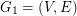and its first arbitrary shortest spanning tree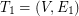. We define the next graphand find onthe second arbitrary shortest spanning tree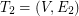. We continue similarly by finding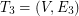on, etc. Let k be the smallest number of disjoint shortest spanning trees as defined above and letbe the graph obtained as union of alldisjoint trees.

Question 1. What is the smallest number of disjoint spanning trees creates a graph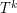containing a Hamiltonian path.

Question 2. What is the smallest number of disjoint spanning trees creates a graphcontaining a shortest Hamiltonian path?

Questions 3 and 4. Replace in questions 1 and 2 a shortest spanning tree by a 1-tree. What is the smallest number of disjoint 1-trees creates a Hamiltonian graph? What is the smallest number of disjoint 1-trees creates a graph containing a shortest Hamiltonian cycle?

Keywords: 1-trees; cycle; Hamitonian path; spanning trees

Bigger cycles in cubic graphs ★★

Author(s):

Problem   Letbe a cyclically 4-edge-connected cubic graph and letbe a cycle of. Must there exist a cycleso that?

Keywords: cubic; cycle

Antichains in the cycle continuous order ★★

Author(s): DeVos

If,are graphs, a function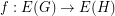is called cycle-continuous if the pre-image of every element of the (binary) cycle space ofis a member of the cycle space of.

Problem   Does there exist an infinite set of graphsso that there is no cycle continuous mapping betweenandwhenever?

Keywords: antichain; cycle; poset

Hamiltonian paths and cycles in vertex transitive graphs ★★★

Author(s): Lovasz

Problem   Does every connected vertex-transitive graph have a Hamiltonian path?

Keywords: cycle; hamiltonian; path; vertex-transitive

Decomposing eulerian graphs ★★★

Author(s):

Conjecture   Ifis a 6-edge-connected Eulerian graph and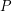is a 2-transition system for, then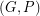has a compaible decomposition.

Keywords: cover; cycle; Eulerian

Faithful cycle covers ★★★

Author(s): Seymour

Conjecture   If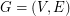is a graph,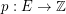is admissable, and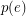is even for every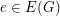, then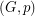has a faithful cover.

Keywords: cover; cycle

(m,n)-cycle covers ★★★

Author(s): Celmins; Preissmann

Conjecture   Every bridgeless graph has a (5,2)-cycle-cover.

Keywords: cover; cycle

The circular embedding conjecture ★★★

Author(s): Haggard

Conjecture   Every 2-connected graph may be embedded in a surface so that the boundary of each face is a cycle.

Keywords: cover; cycle

Cycle double cover conjecture ★★★★

Author(s): Seymour; Szekeres

Conjecture   For every graph with no bridge, there is a list of cycles so that every edge is contained in exactly two.

Keywords: cover; cycle